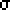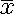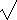# Estimation and Confidence Intervals

### Point and Interval Estimates

Recall how the critical value(s) delineated our region of rejection. For a two-tailed test the distance to these critical values is also called the margin of error and the region between critical values is called the confidence interval. Such a confidence interval is commonly formed when we want to estimate a population parameter, rather than test a hypothesis. This process of estimating a population parameter from a sample statistic (or observed statistic) is called statistical estimation. We can either form a point estimate or an interval estimate, where the interval estimate contains a range of reasonable or tenable values with the point estimate our "best guess." When a null hypothesis is rejected, this procedure can give us more information about the variable under investigation. It can also test many hypotheses simultaneously. Although common in science, this use of statistics may be underutilized in the behavioral sciences.

### Confidence Intervals/Margin of Error

The value=/n is often termed the standard error of the mean. It is used extensively to calculate the margin of error which in turn is used to calculate confidence intervals.

Remember, if we sample enough times, we will obtain a very reasonable estimate of both the population mean and population standard deviation. This is true whether or not the population is normally distributed. However, normally distributed populations are very common. Populations which are not normal are often "heap-shaped" or "mound-shaped". Some skewness might be involved (mean left or right of median due to a "tail") or those dreaded outliers may be present. It is good practice to check these concerns before trying to infer anything about your population from your sample.

Since 95.0% of a normally distributed population is within 1.96 (95% is within about 2) standard deviations of the mean, we can often calculate an interval around the statistic of interest which for 95% of all possible samples would contain the population parameter of interest. We will assume for the sake of this discussion that this statistic/parameter is the mean.
 The margin of error is the standard error of the mean,/n, multiplied by the appropriate z-score (1.96 for 95%).

 A 95% confidence interval is formed as: estimate +/- margin of error.

We can say we are 95% confident that the unknown population parameter lies within our given range. This is to say, the method we use will generate an interval containing the parameter of interest 95% of the time. For life-and-death situations, 99% or higher confidence intervals may quite appropriately be chosen. The population parameter either is or is not within the confidence interval so we must be careful to say we have 95% confidence that it is within, not that there is a 95% probability that it is. Since we expect it to 95% of the time, this can be a point of confusion.

Example: Assume the population is the U.S. population with a mean IQ of 100 and standard deviation of 15. Assume further that we draw a sample of n=5 with the following values: 100, 100, 100, 100, 150. The sample mean is then 110 and the sample standard deviation is easily calculated as sqrt((102+102+102+102+402)/(5-1)) =sqrt(500) or approximately 22.4. The standard error of the mean is sqrt(500)/sqrt(5)=sqrt(100)=10. Our 95% confidence intervals are then formed with z=+/-1.96. Thus based on this sample we can be 95% confident that the population mean lies betwen 110-19.6 and 110+19.6 or in (90.4,129.6). Suppose, however, that you did not know the population standard deviation. Then since this is also a small sample you would use the t-statistics. The t-value of 2.776 would give you a margin of error of 27.8 and a corresponding confidence interval of (82.2, 137.8).

### Finite Population Correction Factor

 The finite population correction factor is:((N-n)/(N-1)).

If you are sampling without replacement and your sample size is more than, say, 5% of the finite population (N), you need to adjust (reduce) the standard error of the mean when used to estimate the mean by multiplying it by the finite population correction factor as specified above. If we can assume that the population is infinite or that our sample size does not exceed 5% of the population size (or we are sampling with replacement), then there is no need to apply this correction factor.

### Known vs. Unknown Population Variance

There is a pervasive joke in inferential statistics about knowing the population variance or population standard deviation. If such a value were known, then we have a big handle on how the population is distributed and would seem to have little reason to do inferential statistics on a sample. However, there are times when a test, like an IQ test, might be designed with a given variance in mind and such an assumption is meaningful.

The only practical difference is that unless our sample size is large enough (n > 30) we should use the more conservative t distribution rather than the normal distribution to obtain the critical value of our test statistic when the population variance (standard deviation) is unknown and also use s2 (or s) as an estimate of the population variance (or standard deviation) when calculating the standard error of the mean or t value.

### Statistical Precision

Statistical Precision can be thought of as how narrow our margin of error is. For increased precision a larger sample size is required. However, the precision increases slowly due to the square root of n in the denominator of the formula. Thus to cut a margin of error in half would require one to increase the sample size by a factor of four. Of course, the margin of error is also influenced by our level of significance or confidence level, but that tends to stay fixed within a field of study. A 99% confidence interval will be wider than a 95% confidence interval or less precise. The same basic situation applies for the correlation coefficient and population proportion tests described below even though different formulae determine our test statistic.

### Testing rho=a (Correlation Coefficient): Fisher z

Sociologists might commonly test hypotheses regarding the correlation between two variables or construct an interval estimate of such a correlation. However, a transformation of variable is necessary since the sampling distribution is skewed when there is a correlation. The special case of testing for no correlation will be handled with a normal distribution in the next section.

The Fisher z transformation transforms the correlation coefficient r into the variable z which is approximately normal for any value of r, as long as the sample size is large enough. However, the transformation goes beyond simple algebra so a conversion table is included in the Hinkle text. We don't expect to test over this material so this is included here only for reference.

The transformation involves the logarithm function which relates a given number (y) to another number (x) which is the exponent required to raise some base (b) to, to obtain the given number (y = bx). The usual base used is that of the natural logarithm or base e = 2.71828... (It can also be described as the hyperbolic cotangent function.)

zr=½log((1+r)/(1-r)).

The standard error of zr is given by

szr=sqrt(1/(n-3)).

n, of course, is the sample size. We then procede with hypothesis testing or confidence interval construction by forming the test statistic in the usual manner of (statistic-parameter)/standard error of the statistic. It is usual to call the population correlation coefficient rho.

### Testing rho=0 (Correlation Coefficient)

A common test in the behavioral sciences is that of whether or not a relationship exists between two variables. Zero correlation in a population is a special case where the t distribution can be used after a slightly different transformation.

t=r•sqrt((n-2)/(1-r2)).

n is our usual sample size and n-2 the degrees of freedom (with one lost for [the variance of] each variable).

Example: Consider a two-tailed test to check H0: rho=0 at alpha=0.05 for a sample of 22 ordered pairs when r=0.45.
Solution: The critical t values are +/-2.086. t=0.45•sqrt((22-2)/(1-0.452))=2.254. We can thus reject the null hypothesis or as commonly stated find the relationship to be statistically significant. We can construct a corresponding confidence interval, which should be done using the Fisher z transformation of the previous section (since rho#0): r=0.45 transforms to zr=0.485 and szr=0.229. The 95% confidence interval is then zr+/-1.96•0.229 = 0.485+/-0.450 or (0.035,0.935). Note that these are still z scores which transform back to (0.035,0.733) as r values. (The inverse transformation might easiest be done with a table of values or via the time honored guess and check method, instead of using the inverse hyperbolic cotangent.) The transformation equation above is commonly avoided by the use of tables compiled to give critical r values for various sample sizes and alphas.

### Testing P=a (Population Proportion)

An uppercase P is used for population proportion since the Greek letter pi almost always refers to the ratio of a circle's circumference to its diameter (3.1415...).

When two possibilities exist for a particular variable in a population, the binomial distribution provides an easily identifiable standard error of the proportion in terms of p, the hypothetical proportion value, q=1 - p, and the sample size n. Since the binomial tends toward the normal distribution quickly we can use the normal distribution when np AND nq both exceed some magic number, say 10. Some less conservative disciplines might even push that magic number down to 5, whereas more conservative disciplines push it up to 15. This magic number check helps ensure adequately sized samples when p takes on values far away from ½, i.e. near either 0 or 1.

The formula for the standard error of the proportion is:     sp = sqrt(pq/n).

(Take care here not to assume you can find this by dividing the standard deviation for a binomial distribution by the square root of the sample size!)

Example: Suppose 35% of first-year college students applied to some other college than where they are actually attending. Suppose further that you will be asking a simple random sample of size n = 1000 from the population of about N = 1,600,000 and desire a result within 3% of the true value. How probable is this?
Solution: We expect a mean sample proportion of p = 0.35 distributed normally with a standard deviation of sqrt(pq/n) = 0.0151. We can calculate P(0.32 < p < 0.38) = P(-1.989 < z < 1.989) = 0.953 or slightly more than 95% of all samples will give such a result.

We should note that the confidence interval constructed about our test statistic using the hypothesized population parameter and the confidence interval constructed using the actual sample statistic will differ. (See Hinkle page 229.)